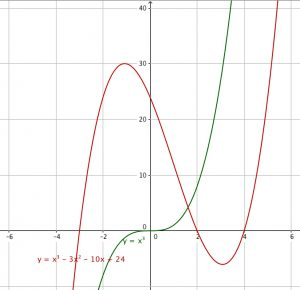Lesson Activities Forums Teacher’s Discussion Forum INTERPRETATION OF CUBIC GRAPHS

• Author
Posts
• #2493Learners must sketch cubic graphs in Grade 10 term 2. The simplest cubic function is f(x) = x^3 which is always increasing and flat at the origin.

This is the graph of the cubic function f(x)= x^3 and the graph of the cubic function

g(x)= x^3 – 3x^2 – 10x + 24

which can also be written as

g(x) = (x + 3)(x – 2)(x – 4)

This graph cuts the x axis at x = -3, x = 2 and x = 4.

To find the turning points of a graph you find the values of x where the derivative is zero. As the derivative of a cubic polynomial is a quadratic polynomial, putting this equal to zero gives two solutions so a cubic graph has one maximum and one minimum point and can cut the x axis at one point or at 3 points.

Remember that graphs of polynomials, trig and exponential functions are smooth curves.

Is there any specific question you want to ask about cubic polynomials?

• #2831

When it comes to interpretation, you must know that roots must three or less but in all essence they must be three. The derivative of a cubic function is a quadratic function as well as derivative of a quadratic is a linear function.

• #9341

Thank you Toni, that was helpful

• #9348

thankyou

• #9346

Your information was of great importance and very helpful.

• #9342

Thankyou Toni

You must be logged in to reply to this topic.

### One Response to INTERPRETATION OF CUBIC GRAPHS

1.Dumisani Xoko says:

I so wish to know probability I have been teaching grade 12 for most of teaching experience. I need help for especially with independent and dependent invents